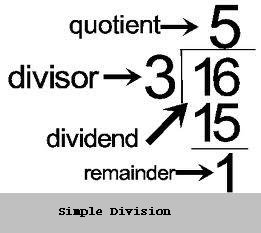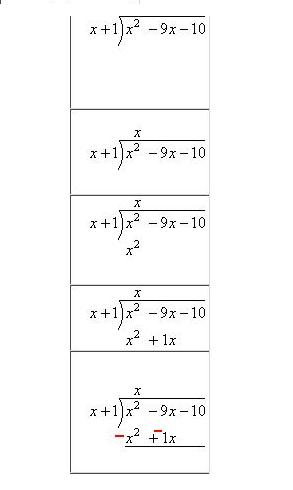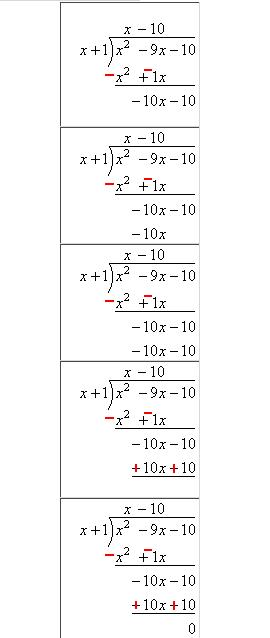# How to Do Long Division

As the students progress in their academics their difficulty level also increases at the same time. With the exception for a few traditional things, the studies keep on getting hard. If we talk in terms of the subjects, it is Mathematics that is likely to become tougher with the up-gradation perhaps more than any other subjects. There are many students who happen to have a real hard time while doing division sums. For this reason, they are hardly able to breathe when they come across situations like solving sums related to long divisions. If the long division involves polynomials or algebraic expressions, than many such students are all at sea. Nevertheless, there are a few things which can make life easy while handling sums pertaining long division.

### Instructions

• 1

First of all remember the rule for divisibility for example, a number is divisible by 2 if the last number is zero or even and with 3 if the sum of total digits is a divisible by 3. Similarly, a number is divisible by 5 if the last number is 0 or 5.

• 2

While solving long division involving digits, consider the divisor and the dividend. If the divisor is a 2-digit number and the dividend has more digits then multiply divisor with such a number that gives a figure which is close to the first two or three digits of the divisor but should be equal or smaller.• 3

Once you have come with a number equal or close to that of the dividend, make subtraction and continue to solve the rest of the digits in the same way until you have a zero or a number smaller than the divisor as remainder.

• 4

Similarly, to solve long divisions involving polynomials or Algebraic expressions such as x2 – 9x – 10 divided by x + 1, multiply the divisor with ‘x’ as it will form an expression with the power equal to that of the dividend such as x2 + x.• 5

Changing the sign in the next step you will have the expression -10 x – 10. Multiplying x + 1 again by -10 will give an expression that will be simplified to zero as shown in the image.•